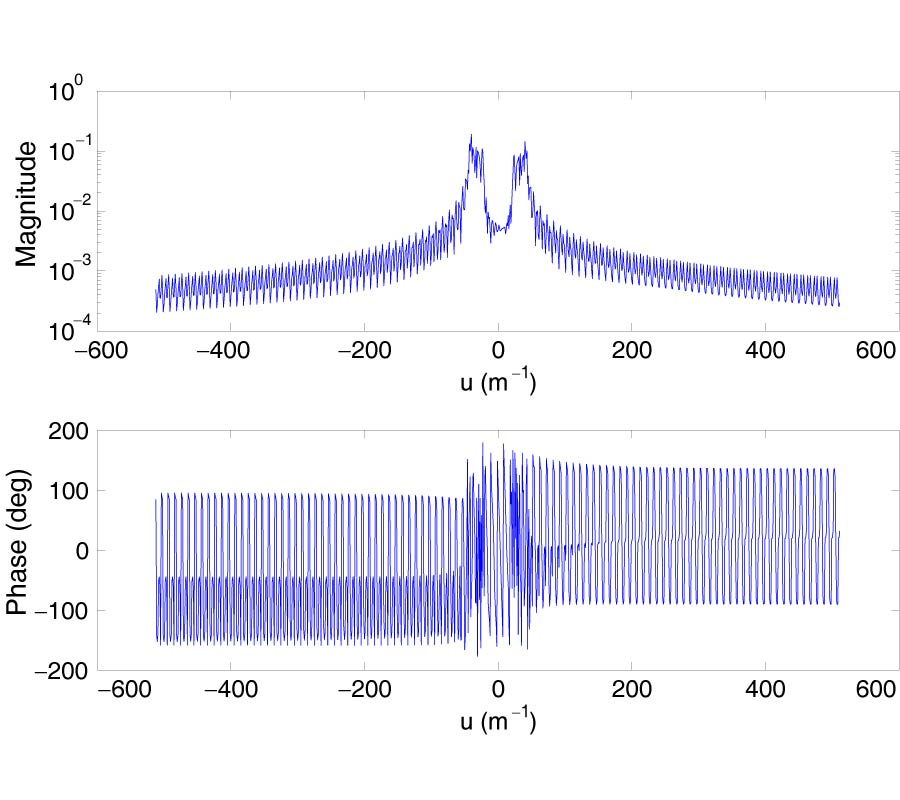# Vol. 109

Front:[PDF file] Back:[PDF file]
Latest Volume
All Volumes
All Issues
2010-11-06

#### A High Accuracy Conformal Method for Evaluating the Discontinuous Fourier Transform

By Chun-Hui Zhu, Qing Huo Liu, Yi Shen, and Lijun Liu
Progress In Electromagnetics Research, Vol. 109, 425-440, 2010
doi:10.2528/PIER10082007

## Abstract

A highly accurate, fast algorithm is proposed to evaluate the finite Fourier transform of both continuous and discontinues functions. As the discretization is conformal to the function discontinuities, this method is called the conformal Fourier transform (CFT) method. It is applied to computational electromagnetics to calculate the Fourier transform of induced electric current densities in a volume integral equation. The spectral discrimination in the CFT method can be arbitrary and the spectral range can be as large as needed. As no discretization for the Fourier exponential kernel is needed, the CFT method is not restricted by the Nyquist sampling theorem, thus avoiding the aliasing distortions that exist in other traditional methods. The accuracy of the CFT method is greatly improved since the method is based on high order interpolation and the closed-form Fourier transforms for polynomials partly reduce the error due to discretization. Assuming Ns and N are the numbers of sampling points in the spatial and frequency domain, respectively, the computational cost of the CFT method is O((M + 1)N log2L), where M is the interpolation order and L=(Ns−1)/M. Applications in spectral analysis of electromagnetic fields are demonstrated.

## Citation

Chun-Hui Zhu, Qing Huo Liu, Yi Shen, and Lijun Liu, "A High Accuracy Conformal Method for Evaluating the Discontinuous Fourier Transform," Progress In Electromagnetics Research, Vol. 109, 425-440, 2010.
doi:10.2528/PIER10082007
http://jpier.org/PIER/pier.php?paper=10082007

## References

1. Liu, Y. H., Z. P. Nie, and Q. H. Liu, "DIFFT: A fast and accurate algorithm for Fourier transform integrals of discontinuous functions," IEEE Microwave and Wireless Components Letters, Vol. 18, No. 2, 716-718, 2008.
doi:10.1109/LMWC.2008.2005162

2. Fan, Z., R. S. Chen, H. Chen, and D. Z. Ding, "Weak form nonuniform fast Fourier transform method for solving volume integral equations," Progress In Electromagnetics Research, Vol. 89, 275-289, 2009.
doi:10.2528/PIER08121308

3. Semnani, A. and M. Kamyab, "Truncated cosine Fourier series expansion method for solving 2-D inverse scattering problems," Progress In Electromagnetics Research, Vol. 81, 73-97, 2008.
doi:10.2528/PIER07122404

4. Huang, Y., Y. Liu, Q. H. Liu, and J. Zhang, "Improved 3-D GPR detection by NUFFT combined with MPD method," Progress In Electromagnetics Research, Vol. 103, 185-199, 2010.
doi:10.2528/PIER10021005

5. Yang, S., Y. Chen, and Z. P. Nie, "Simulation of time modulated linear antenna arrays using the FDTD method," Progress In Electromagnetics Research, Vol. 98, 175-190, 2009.
doi:10.2528/PIER09092507

6. Najjar-Khatirkolaei, B. N. and A. R. Sebak, "Slot antenna on a conducting elliptic cylinder coated by nonconfocal chiral media," Progress In Electromagnetics Research, Vol. 93, 125-143, 2009.
doi:10.2528/PIER09033003

7. Liu, Y., Z. Liang, and Z. Yang, "Computation of electromagnetic dosimetry for human body using parallel FDTD algorithm combined with interpolation technique," Progress In Electromagnetics Research, Vol. 82, 95-107, 2008.
doi:10.2528/PIER08021603

8. Swillam, M. A., M. H. Bakr, and X. Li, "Full wave sensitivity analysis of guided wave structures using FDTD," Journal of Electromagnetic Waves and Applications, Vol. 22, No. 16, 2135-2145, 2008.
doi:10.1163/156939308787522474

9. Lei, J. Z., C. H. Liang, W. Ding, and Y. Zhang, "EMC analysis of antennas mounted on electrically large platforms with parallel FDTD method," Progress In Electromagnetics Research, Vol. 84, 205-220, 2008.
doi:10.2528/PIER08071303

10. Froeyen, M. and L. Hellemans, "Improved algorithm for the discrete Fourier transform," Review of Scientific Instruments, Vol. 56, 2325, 1985.
doi:10.1063/1.1138528

11. Beaudoin, N. and S. S. Beauchemin, "A new numerical Fourier transform in d-dimensions," IEEE Transactions on Signal Processing, Vol. 51, No. 5, 1422-1430, 2003.
doi:10.1109/TSP.2003.810285

12. Sundararajan, D., The Discrete Fourier Transform: Theory, Algorithms and Applications, World Scientific Pub. Co. Inc., 2001.
doi:10.1142/9789812810298

13. Rabiner, L. R., R. W. Schafer, and C. M. Rader, "The chirp z-transform algorithm and its application," IEEE Trans. Audio Electroacoust, Vol. 17, 86-92, 1969.
doi:10.1109/TAU.1969.1162034

14. Simonen, P. and H. Olkkonen, "Fast method for computing the Fourier integral transform via Simpson's numerical integration," Journal of Biomedical Engineering, Vol. 7, No. 4, 337-340, 1985.
doi:10.1016/0141-5425(85)90067-6

15. Zeng, P., "High-accuracy formula for discrete calculation of fourier transforms," Applied Mathematics and Computation, Vol. 106, No. 2-3, 117-140, 1999.
doi:10.1016/S0096-3003(98)10080-2

16. Morelli, E. A., "High accuracy evaluation of the finite Fourier transform using sampled data," NASA TM, Vol. 110340, 1997.

17. Fan, G.-X. and Q. H. Liu, "Fast Fourier transform for discontinuous functions," IEEE Transactions on Antennas and Propagation, Vol. 52, No. 2, 461-465, 2004.
doi:10.1109/TAP.2004.823965

18. Liu, Y. H., Q. H. Liu, and Z. P. Nie, "A new efficient FDTD time-to-frequency-domain conversion algorithm," Progress In Electromagnetics Research, Vol. 92, 33-46, 2009.
doi:10.2528/PIER09030906

19. Higham, N. J., Accuracy and Stability of Numerical Algorithms, Society for Industrial Mathematics, 2002.
doi:10.1137/1.9780898718027

20. Sarkar, I. and A. T. Fam, "The interlaced chirp z transform," Signal Processing, Vol. 86, No. 9, 2221-2232, 2006.
doi:10.1016/j.sigpro.2005.10.004

21. Graillat, S., P. Langlois, and N. Louvet, "Compensated horner scheme,", Research Report, Vol. 4, 2005.

22. El-Mikkawy, M. E. A., "Explicit inverse of a generalized Vandermonde matrix," Applied Mathematics and Computation, Vol. 146, No. 2-3, 643-651, 2003.
doi:10.1016/S0096-3003(02)00609-4